# 自然语言处理 12：语篇

## 墨尔本大学 COMP90042 课程笔记

Posted by YEY on April 9, 2020

# Lecture 12 语篇

## 1. 语篇

### 1.1 语篇

• 目前为止，我们学习的大部分任务/模型都是在 单词或者句子层面 操作的：
• 词性标注：通常每次标注一个句子中所有单词的词性。
• 语言模型：从 n-grams 语言模型到 RNN 语言模型，它们都是在句子层面操作的。
• 词汇/分布语义学：当我们训练这些模型时通常也是以句子作为边界的。
• 但是，NLP 也会经常需要处理 文档
• 语篇（Discourse）：理解文档中的句子之间是如何关联起来的。因此，当我们浏览一个文档时，语篇为我们提供了一个关于该文档的连贯的故事线。

### 1.2 三个关键的语篇任务

• 语篇分段（Discourse segmentation）
例如：我们有一篇文章，我们希望将它按照内容之间的连贯性分成一些独立的块（chunks）。例如，第一段是关于文章摘要（Abstract）的，第二段是关于文章内容介绍（Introduction）的，因此，我们希望在这两个段落之间插入一个分隔。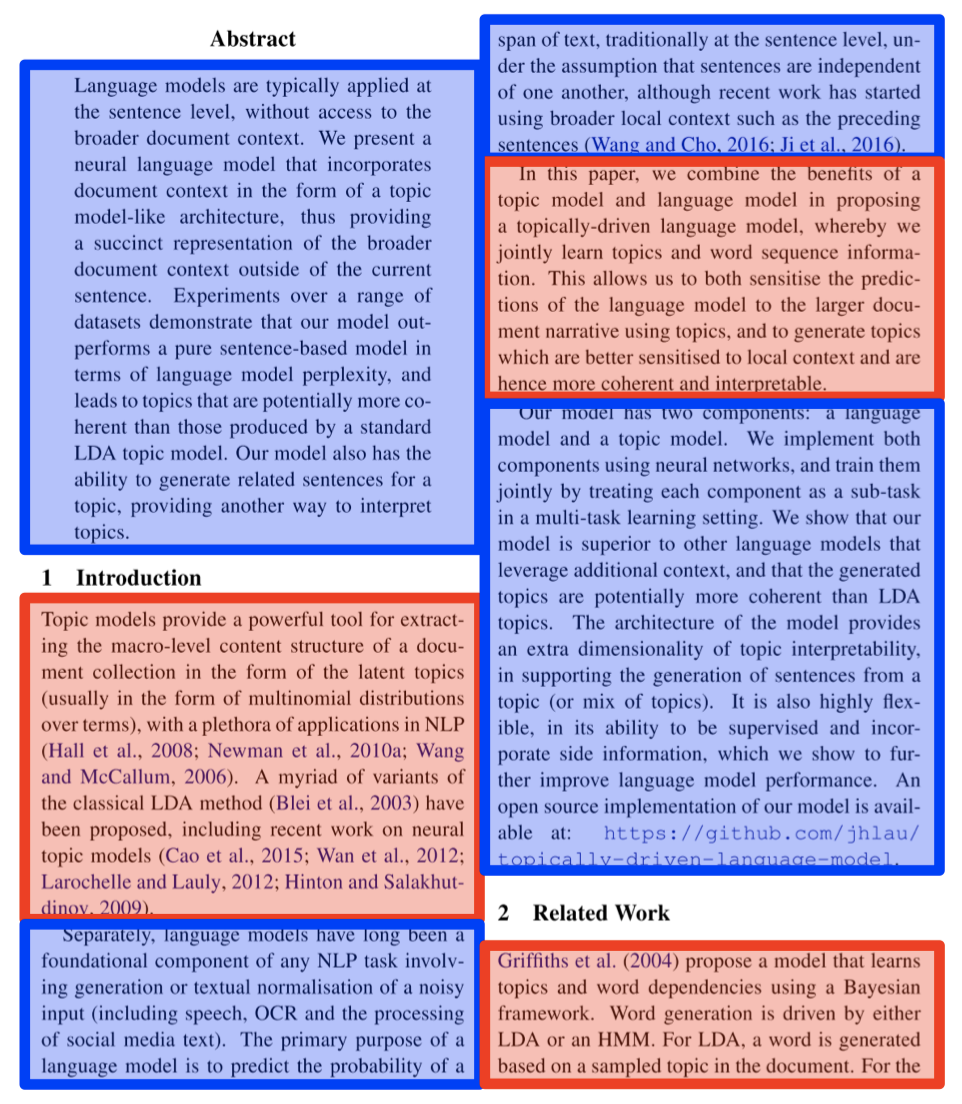• 语篇解析（Discourse parsing）
语篇解析的核心思想是试图将文档组织为一种 层级结构（hierarchical structure）。例如：我们有一个包含 3 个句子的非常小的文档，语篇解析试图将这 3 个彼此关联的子句 (clauses) 组织为一个层级树形结构。可以看到，这段文本的中心句是第一个子句，后面的两个子句只是用来支持前面句子的观点的。因此，这三个子句被组织为下面的树形结构。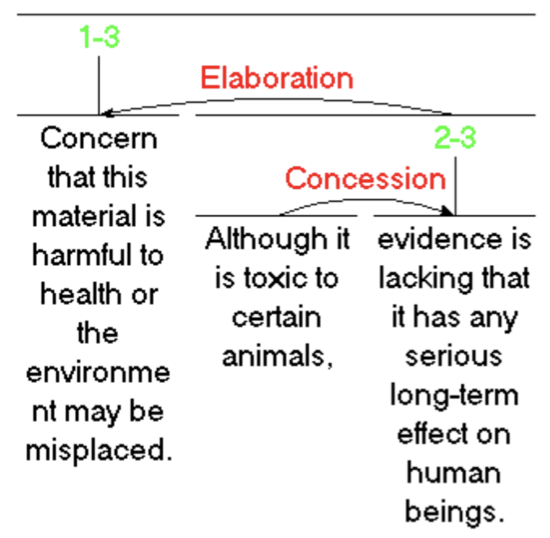• 指代消解（Anaphora resolution）
指代消解的目的是关于消除文档中的指代词的歧义问题。例如：在下面的句子中，代词 “$\textit{He}$” 在不同上下文中的指代对象是谁。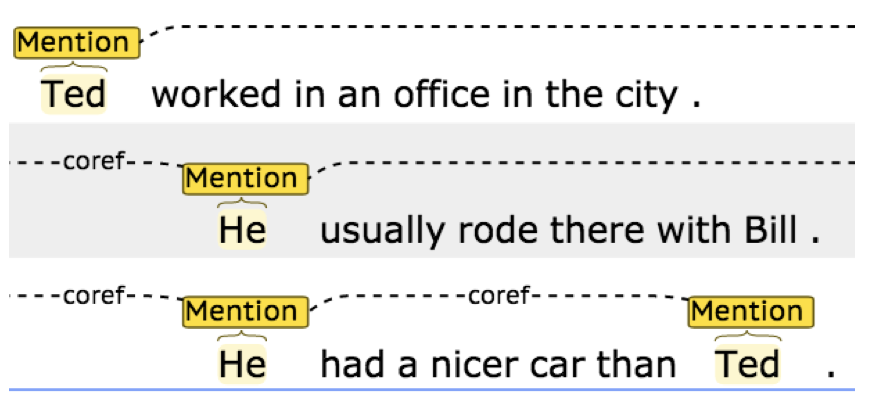## 2. 语篇分段

### 2.1 语篇分段

• 一个 文档（document）可以被视为 一个由分段组成的序列（a sequence of segments）
• 分段（Segment）：一段连贯的文字。
• 连贯性（Cohesion）
• 连贯性意味着这段文字是围绕某个特定 主题（topic）功能（function）来组织的。
• 维基百科里的人物类传记：早年经历 (early years)、主要事件 (major events)、其他方面的影响 (impact on others)
• 科学性的文章：简介 (introduction)、相关工作 (related work)、实验 (experiments)

### 2.2 无监督方法

• TextTiling 算法：寻找句子之间具有较低词汇连贯性的点。
• 对于每个句子间隙（sentence gap）：
• 创建两个 词袋向量（BOW vectors），它们由间隙两侧的各自 $k$ 个句子中的单词组成。
• 计算两个向量的余弦相似度得分。
• 对于间隙 $i$，计算一个 深度分数（depth score），当深度分数超过某个 阈值（threshold）$t$ 时，就在这个间隙处插入一个分界线。

$\text{depth}(gap_i)=(sim_{i-1}-sim_{i})+(sim_{i+1}-sim_{i})$

### 2.3 TextTiling 的例子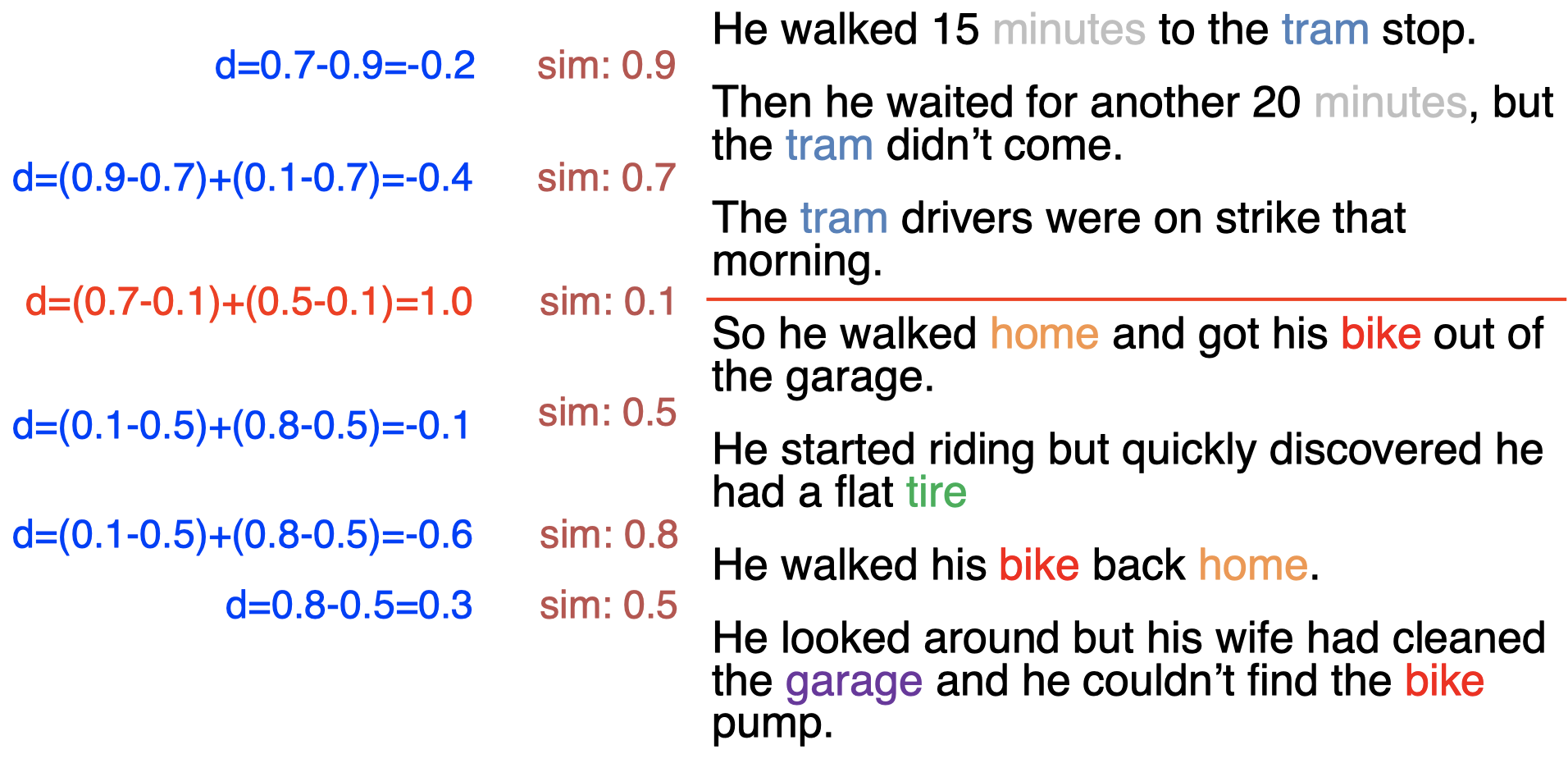$\text{depth}(gap_i)=(sim_{i-1}-sim_{i})+(sim_{i+1}-sim_{i})$

### 2.4 有监督方法

• 我们可以从一些容易获得的渠道得到一些带标签数据：
• 科学出版物
• 维基百科的文章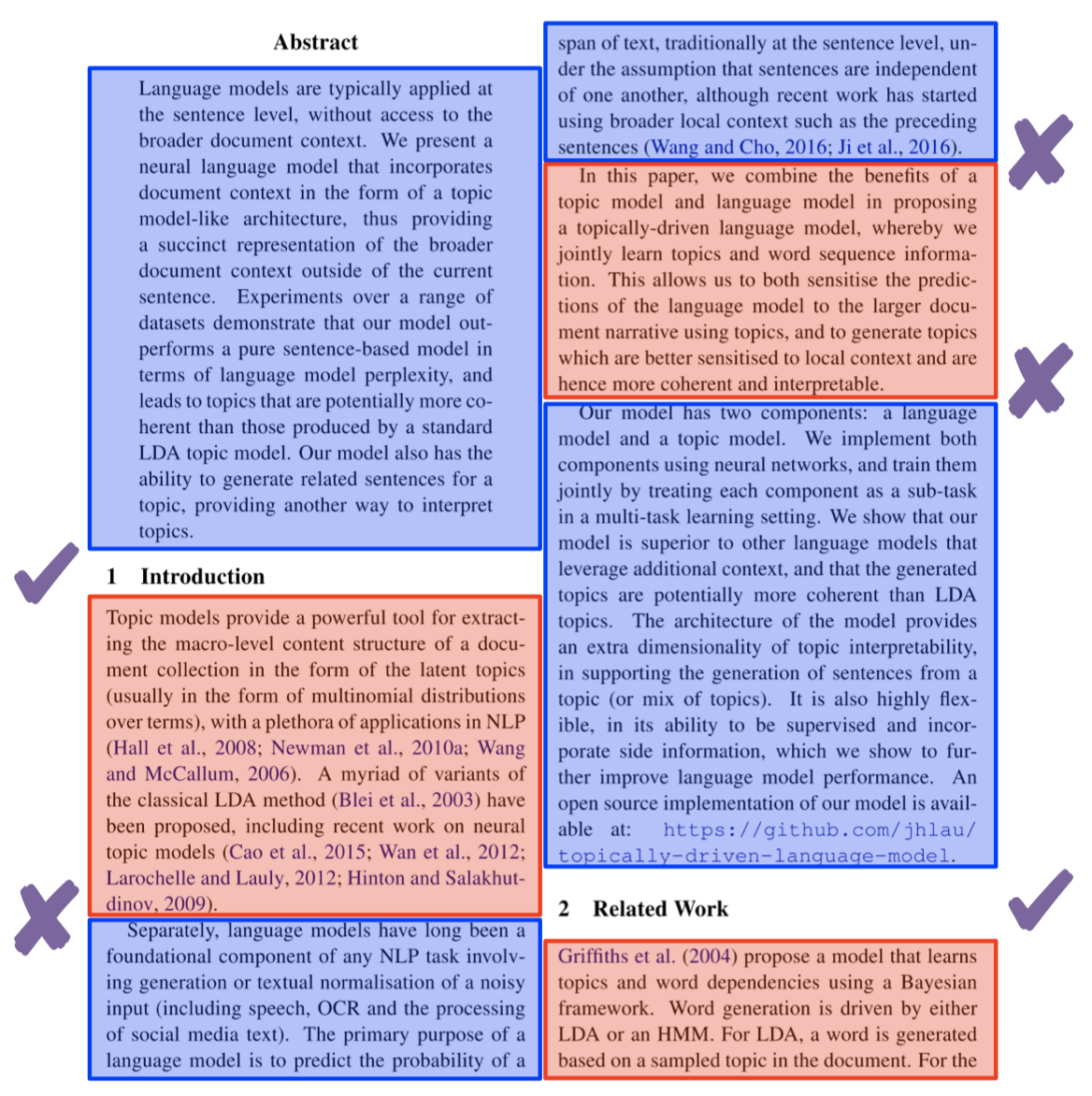### 2.5 有监督语篇分段器

• 应用一个二分类器来识别边界。
就像前面提到的例子，我们可以采用一个基于正负标签数据的二分类器来决定是否需要对给定的两个段落进行切分。

• 或者使用序列分类器。
我们也可以使用像 HMM 或者 RNN 这类序列模型进行分类。这种情况下，我们在分段时会考虑一些上下文信息，从而在分段时得到一个全局最优的决策结果。

• 我们还可以潜在地包含分类的章节类型 (section type)，例如：Introduction, Conclusion 等。
假如我们使用维基百科或者科学文章，我们知道其中每个章节都有特定的主题/功能，我们可以原问题转换为一个多任务问题：我们不仅对语篇文本进行分段，并且我们还需要给出每个分段所对应的章节。

• 我们还可以集成一些更宽泛的特征，包括：

• 分布语义学
• 语篇标记（discourse markers），例如：$\textit{therefore, and, however}$ 等。
语篇标记在这里要更加重要一些，因为它们通常对于语篇分段前后的差异具有放大效应。

## 3. 语篇解析

### 3.1 语篇解析

• 识别 语篇单元 (discourse units)，以及它们之间所维系的 关系（relations）
• 修辞结构理论 (Rhetorical Structure Theory, RST) 是一个对文档中的语篇结构进行层级分析的框架。RST 在计算机科学中具有广泛应用，例如：总结 (Summarisation)、问答 (QA) 等。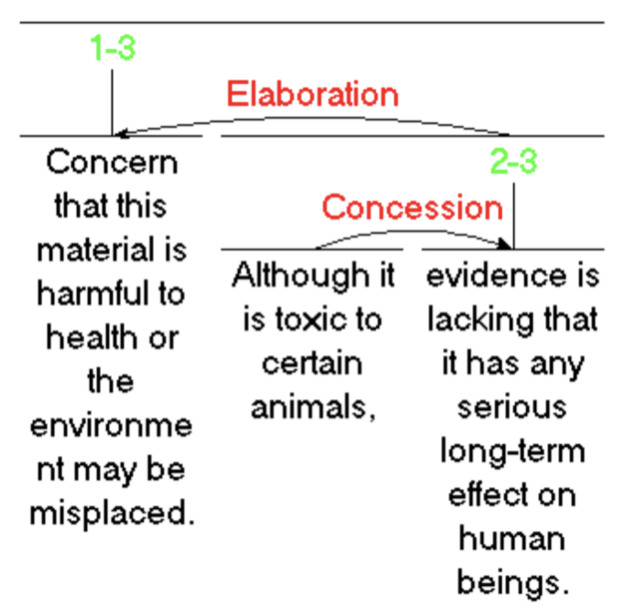### 3.2 RST

• 基本单元：基本语篇单元（elementary discourse units, EDUs）
• 通常是组成一个句子的 子句（clauses）
• EDUs 不会跨越句子边界。 例如，RST 将下面的句子划分为两个 EDUs：• RST 还定义了语篇单元之间的 关系（relations）:
• 连接 (conjuction)，论证 (justify)，让步 (concession)，详述 (elaboration) 等。

例如，在之前的例句中，第二个 EDU 和第一个 EDU 之间是详述 (elaboration) 关系。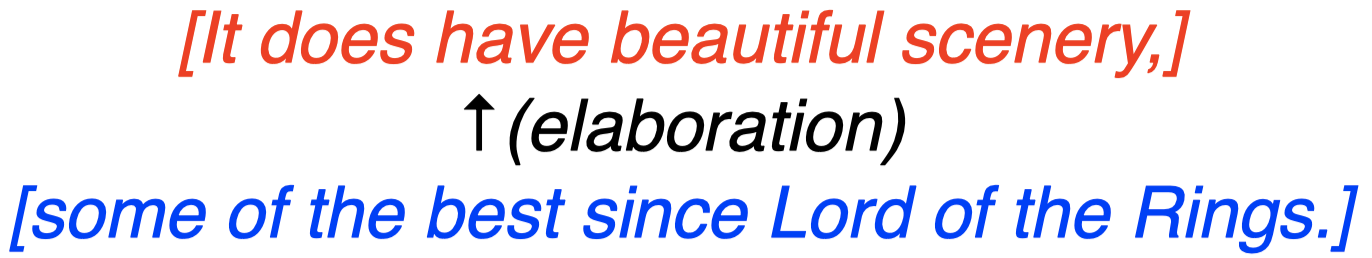### 3.3 核心 vs. 伴随体

• 在每个 RST 语篇关系中，都有一个论点作为 核心（nucleus），即主要论点。
• 支持论点作为 伴随体（satellite）

例如：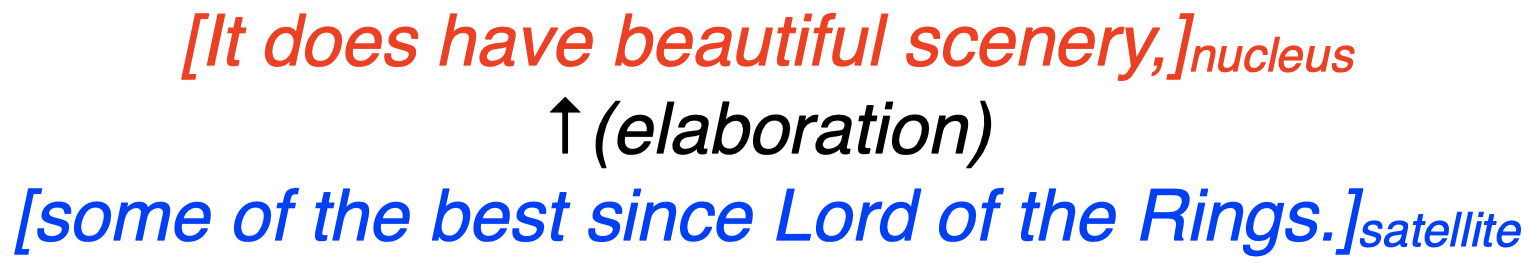• 有些关系是对等的（例如：连接 conjunction），这种情况下，两个论点都是核心（nuclei）。
例如：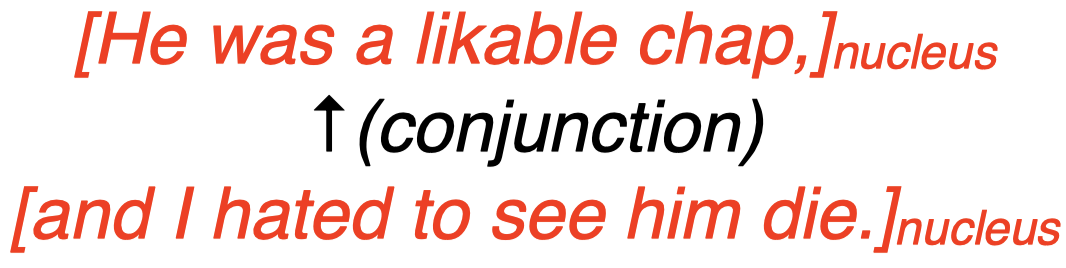### 3.4 RST 树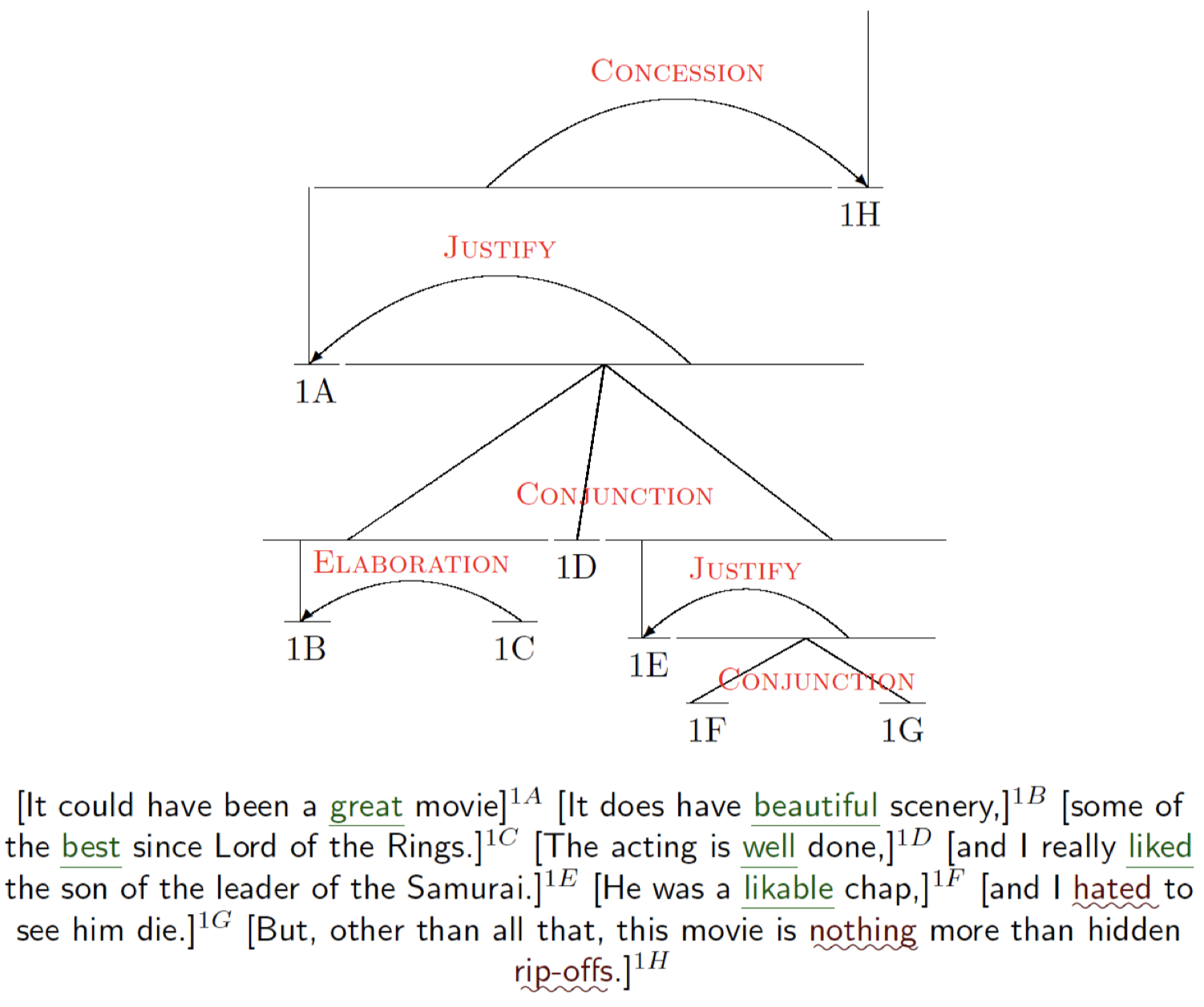• 一段 RST 关系将两个或者更多的语篇单元 (DU) 合并为一个复合语篇单元 (composite DU)。

通常，一个 RST 树的是通过迭代地对语篇单元进行合并的方式构建的。可以看到，每段 RST 关系都是在两个语篇单元之间定义的。例如：$1\text{F}$ 和 $1\text{G}$ 之间存在 $\text{C}\scriptsize{\text{ONJUCTION}}$ 关系，而 $1\text{E}$ 与 $1\text{F}$ 和 $1\text{G}$ 的结合体之间也存在 $\text{J}\scriptsize{\text{USTIFY}}$ 关系。一旦我们将 RST 中的两个语篇单元 (DU) 结合起来，我们将得到一个复合语篇单元 (composite DU)，然后这个复合 DU 就可以和 RST 中的其他 DU 或者复合 DU 形成另外的关系。

• 通过重复合并 DU 的过程来创建一个 RST 树。

在上面的例子中，我们可以自底向上依次对 DU 进行合并，最终得到一个 RST 树。

### 3.5 使用语篇标记进行解析

• 一些 语篇标记 (discourse markers)（提示语）显式地表明了关系。
• 例如：$\textit{although, but, for example, in other words, so, because, in conclusion,}\dots$

这些单词表明了前后语篇单元之间存在某种新的关系。

• 我们可以利用这些语篇标记来构建一个简单的 基于规则的解析器（rule-based parser）

• 例如，我们可以收集所有这些显式的语篇标记，并试图理解其中包含的前后语篇单元之间的关系，然后构建一个简单的基于规则的解析器以用于语篇解析任务。
• 然而：
• 许多关系根本没有用话语标记进行标记。

可能存在这样的情况：某些语篇单元之间并没有显式的语篇标记，但是这些语篇单元之间确实存在某种关系。

• 许多重要的话语标记（例如：$\textit{and}$）具有歧义性（ambiguous）。

• 有时并非用于话语标记
例如：“$\textit{John and I went fishing}$”，这里的 “$\textit{and}$” 连接的只是句子中的两个主语单词，而非两个语篇单元。
• 可能表示多种关系 同一个语篇标记单词可能表示多种关系，例如，“$\textit{and}$” 有时可以表示连接关系（conjunction），有时则表示原因（reason）或者论证（justify）关系。因此，其中存在歧义性问题，我们不能总是基于简单规则将其视为连接关系。

### 3.6 使用机器学习进行解析

• RST 语篇 Treebank
• 包含了超过 300 个带有 RST 树形结构注释的文档。

创建这些文档的成本非常高并且非常耗时，语言学专家需要现将这些文档分成单个的语篇单元，然后缓慢地迭代合并这些语篇单元来构建一个能够描述整篇文档的完整 RST 树。

• 基本思想：
• 对文档进行分段，得到 EDUs。这一步通常比较简单，我们可以构建一个分类器来实现。
• 然后，我们可以构建另一个分类器，来学习如何迭代地将邻接的 DUs 合并为复合 DUs，以构建一个完整的 RST 树。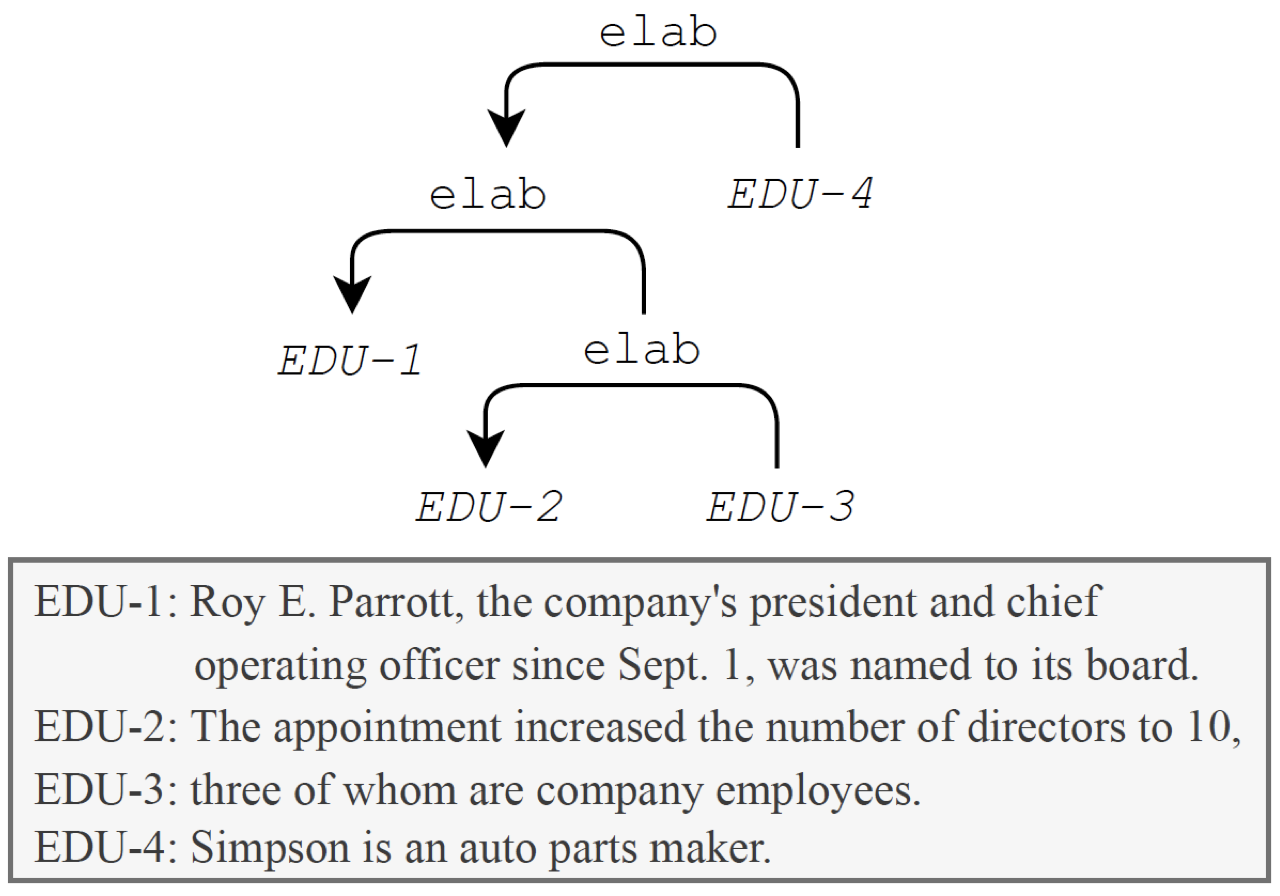例如，这里我们有一个非常简单的文档，它包含 4 个句子。首先，我们构建一个 EDU 分段器，将该文档划分为 4 个 EDUs。然后，我们再构建一个分类器，迭代地对这些 EDUs 进行合并。例如，我们可以自底向上，先对 $\textit{EDU-}2$ 和 $\textit{EDU-}3$ 进行合并，同时我们还需要预测出两者之间的关系 $\text{elab}$。然后，我们按照同样的方式，将两者的复合 DU 与 $\textit{EDU-}1$ 进行合并，并给出新的关系。以此类推，我们可以通过自底向上的方式构建出完整的 RST 树。

• 基于转移的解析（Transition-based parsing）
• 自底向上
• 贪婪方法，使用 shift-reduce 算法。 基于转移的解析可能是语篇解析任务中最常用的方法。该方法一种贪婪方法，类似于局部分类器，这里每个决策点之间都是独立的，它仅仅选择当前最可能的 EDU 对进行合并，而不会不考虑过去和未来的情况。

• CYK / 图解析（chart parsing）算法
• 自底向上
• 全局方法，但是存在一些限制会阻止 CYK 来查找用于语篇解析的全局最优树。
不同于基于转移的解析，CYK 提供的是一种全局方法，但是由于在语篇解析过程中存在一些限制，会阻止 CYK 找到全局最优树，因此，CYK 通常得到的 RST 树并非全局最优。另外，由于该方法要比基于转移的解析方法慢得多，因此，通常在语篇解析任务中，主要采用的方法还是基于转移的解析。

• 自顶向下解析（Top-down parsing）
• 序列标注问题
• BERT另一种使用较少的、更加自动化一点的方法是自顶向下解析。这里，我们将语篇解析视为一个序列标注问题。相比缓慢地自底向上对 EDUs 进行合并，我们可以选择自顶向下进行。我们有一个完整的文档，给定所有的这些 EDUs，现在我们希望自顶向下地寻找相应的切分点。例如，我们首先对 $\textit{EDU-}3$ 和 $\textit{EDU-}4$ 进行切分，将整个语篇文本分为两部分：$\textit{EDU-}1$、$\textit{EDU-}2$、$\textit{EDU-}3$ 作为整体，以及单独的 $\textit{EDU-}4$。然后，对于前者，我们再次寻找切分点，然后对 $\textit{EDU-}1$ 和 $\textit{EDU-}2$ 进行切分。以此类推，我们可以使用这种分治法 (divide-and-conquer approach) 将整个语篇自顶向下切分，得到 RST 树。

这里实际上是一个序列标注问题，因为实际上这里给定了一个由 EDU 间隙组成的序列，我们对每个间隙进行标注，看是否需要在该间隙处进行切分。这种方法的优点是我们可以应用之前学过的预训练语言模型（例如：BERT）来完成这些分段任务。而之前的自底向上的方法则无法很好地与 BERT 结合起来。

### 3.7 语篇解析特征

• 词袋（Bag of words）
• 显然，单词对于语篇解析非常重要。
• 语篇标记（Discourse markers）
• 语篇标记也非常重要，像 “$\textit{however}$” 这类单词通常显式地表明了语篇单元之间的关系。
• 起始/结束 n-grams
• 通常，我们倾向于在句子开头和结尾使用一些特定的单词短语，它们也包含了一些句子之间逻辑上的转变。
• 文本中的位置
• 文本中的句子之间的语篇关系转变有时和句子在文本中的位置有关。
• 句法特征
• 通常，像词性（part-of-speech）等句法特征通常也包含了一些有用信息。
• 词汇/分布语义学
• 单词含义及上下文信息通常也非常有用。

### 3.8 为什么要进行语篇解析？• 总结（Summarisation）

当语篇解析刚被提出的时候，主要是用于文本总结任务的：给定一段文本，我们希望找出这段文本想要表达的主要观点。假如我们知道了这边文档的 RST 树，我们就可以知道其中哪些语篇单元是核心，哪些是伴随体，以及它们之间的关系，这样，我们就可以很容易地对文章内容进行总结归纳，因为我们知道作为核心的语篇单元（例如：$1\text{B}$）的重要程度总是要比作为伴随体的语篇单元（例如：$1\text{C}$）更高。

• 情感分析（Sentiment analysis）

语篇解析的另一个应用场景是情感分析。这里，我们来看一下 RST 树是如何决定这篇文档的情感倾向的。可以看到，我们用绿色标出了这段文本中所有表示正面情感的单词，然后用棕色标出了所有表示负面情感的单词。如果仅仅观察正负情感单词的出现频率，我们可以会认为这段影评表达的是一种正面情感，因为其中出现的大部分都是表示正面情感的单词。但是，如果我们观察这段文本的 RST 树，我们会发现位于根结点的最核心的语篇单元是 $1\text{H}$，它对应文本中的最后一个句子，这表明我们在对这段文本进行情感分析时，最后一个句子表达的意思才是整段文本的重点。然后，我们会发现这段文本的情感倾向实际上是负面的。因此，语篇解析中的 RST 树可以帮助我们发现文本中的中心句。

• 论据挖掘（Argumentation mining）

语篇解析还可以帮助我们进行论据挖掘任务。RST 树可以帮助我们发现文本中用于支持一些观点的论据，例如：RST 树中的 $\text{J}\scriptsize{\text{USTIFY}}$ 关系。

• 论文评分（Essay scoring）

## 4. 指代消解

### 4.1 照应语

• 照应语（Anaphor）：回过头来引用文本中较早出现过的元素的一种语言表达。

• 照应语在语篇中有一个对应的 先行词（antecedent），先行词通常是一些名词短语，但并非总是如此。

例如：其中，“$\textit{It}$” 指代前面的 “$\textit{Ted was late for work}$” 这一事件，而 “$\textit{his}$” 则指代的是 “$\textit{Ted’s}$”。这里的 “$\textit{It}$” 和 “$\textit{his}$” 都是照应语，因为它们都是指代前面出现过的内容。

• 代词（Pronouns）是最常见的照应语
• 但是还有其他各种照应语
• 指示代词（Demonstratives）
例如：$\textit{that problem}$

### 4.2 先行词约束

• 代词必须在 数量 上与其对应的先行词保持一致。例如，上面的句子中，代词 “$\textit{They}$” 是复数形式，因此，其所指代的对象必须是前面句子开头的 “$\textit{His coworkers}$” 而非 “$\textit{Ted}$”。

• 代词必须在 性别 上与其对应的先行词保持一致。例如，上面的句子中，代词 “$\textit{She}$” 表示女性，因此，其所指代的对象必须是句子开头的 “$\textit{Sue}$” 而非 “$\textit{Ted}$”。

• 代词必须是 反身（reflexive）的，即 “-$\textit{self}$” 形式，如果其先行词和该代词在同一个子句中，并且先行词充当主语。例如，上面第一个句子中，代词 “$\textit{him}$” 指代的并非前面的 “$\textit{Ted}$”，而是另有其人；而第二个句子中的代词 “$\textit{himself}$” 指代的对象才是句子开头充当主语成分的 “$\textit{Ted}$”。

### 4.3 先行词偏好

• 代词对应的先行词应该是距离最近的 (recent)。例如，上面的句子中，代词 “$\textit{it}$” 存在歧义性，它可能指代前面的 “$\textit{the tram}$”、“$\textit{his bike}$” 或者 “$\textit{the garage}$”。但是，结合上下文单词 “$\textit{riding}$”，我们会发现这里的 “$\textit{it}$” 指代的显然不可能是 “$\textit{the garage}$”。然后对于剩下的 “$\textit{the tram}$” 和 “$\textit{his bike}$”，我们可以认为代词 “$\textit{it}$” 更有可能指代的是 “$\textit{his bike}$”，因为二者的距离更近一些。

• 根据语法位置，先行词应该出于突出的 (salient) 位置。

因此，当我们选择先行词时，我们通常遵循以下优先级：

主语（subject）$>$ 宾语（object）$>$ 介词主题（argument of preposition）例如，在上面的句子中，第二句中的 “$\textit{He}$” 更有可能指代的是前一句的主语 “$\textit{Ted}$” 而非宾语 “$\textit{Bill}$”，因为在主语在一个句子中的重要程度要高于宾语。

### 4.4 实体和参照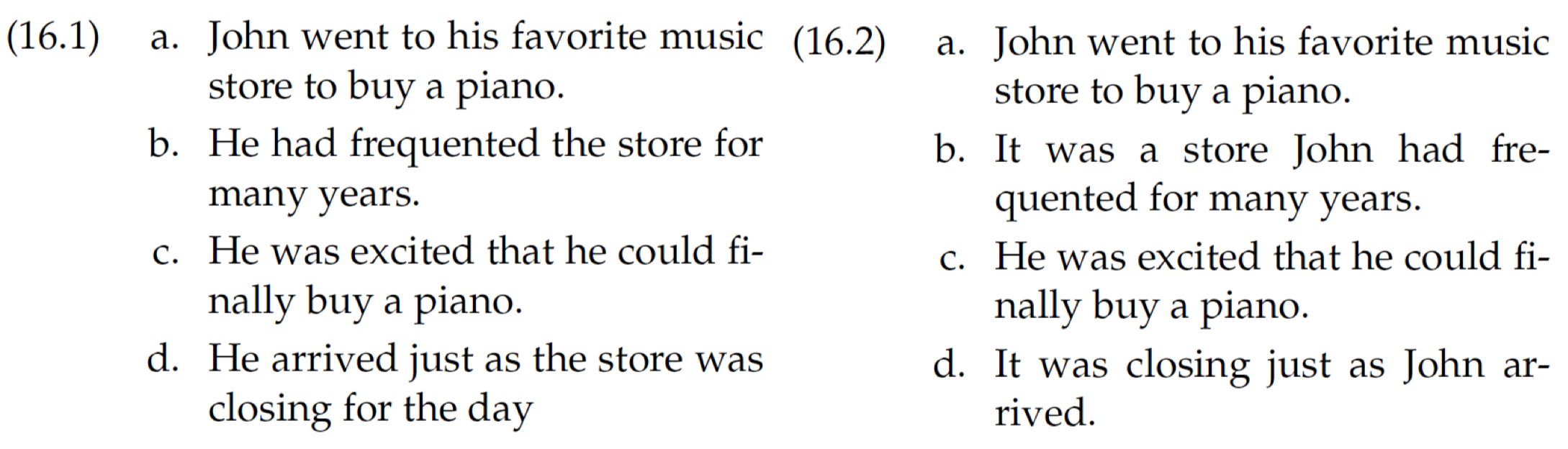• 左边 16.1 的语篇要更具连贯性。
• 因为 16.1 中的所有代词的指代对象都是主人公 John。

### 4.5 中心理论

• 语篇结构与实体参照之间关系的统一描述。

RST 为我们描述了如何表示这些语篇结构，而中心理论要更进一步，它告诉我们如何将语篇结构中的这些实体和参照联系起来。并且，中心理论为我们提供了一种方式来解释为什么之前例子中的 16.1 的语篇要比 16.2 的语篇更具连贯性。

• 语篇中的每个 表述（utterance）都通过一个 实体集（a set of entities）给出，我们将其称为 中心（centers）

• 在解释一些具有歧义性的代词时，我们会偏向于某些特定实体。

### 4.6 表述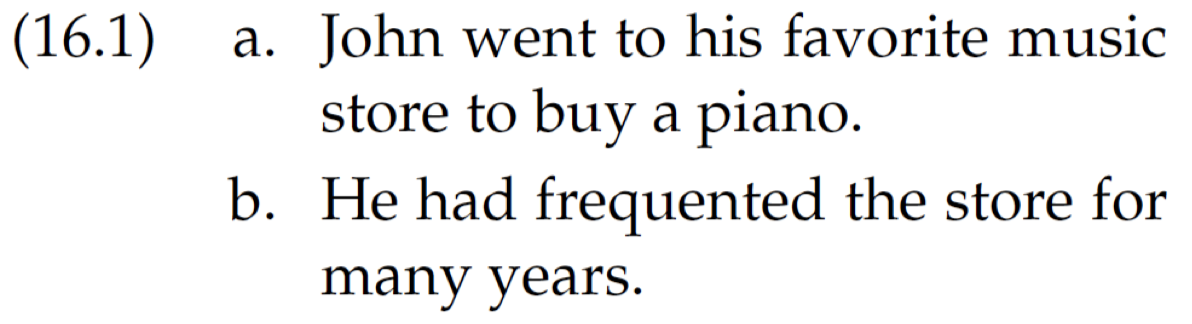• 前向中心（Forward-looking centers）
• 前向中心是指当前表述 $U_n$ 中出现过的所有实体：$C_f(U_n)=[e_1, e_2, \dots]$
• 例如：$C_f(16.1\,a)=[\textit{John, music store, piano}]$
• 按照句法突出性（syntactic prominence）排序：主语（subject）$>$ 宾语（object）
• 后向中心（Backward-looking centers）
• 后向中心是指在前一个表述的前向中心 $C_f(U_{n-1})$ 中排名最高，同时也在当前表述 $U_n$ 中出现过的实体。
• 例如：$C_b(16.1\,b)=[\textit{John} ]$

因为这里前一个表述的前向中心为 $C_f(16.1\,a)=[\textit{John, music store, piano}]$，其中，实体 “$\textit{John}$” 的排名最靠前，然后在当前表述 $16.1\,b$ 中，第一个实体 “$\textit{He}$” 指代的对象也是实体 “$\textit{John}$”。所以当前表述的后向中心为 $C_b(16.1\,b)=[\textit{John} ]$。

### 4.7 中心算法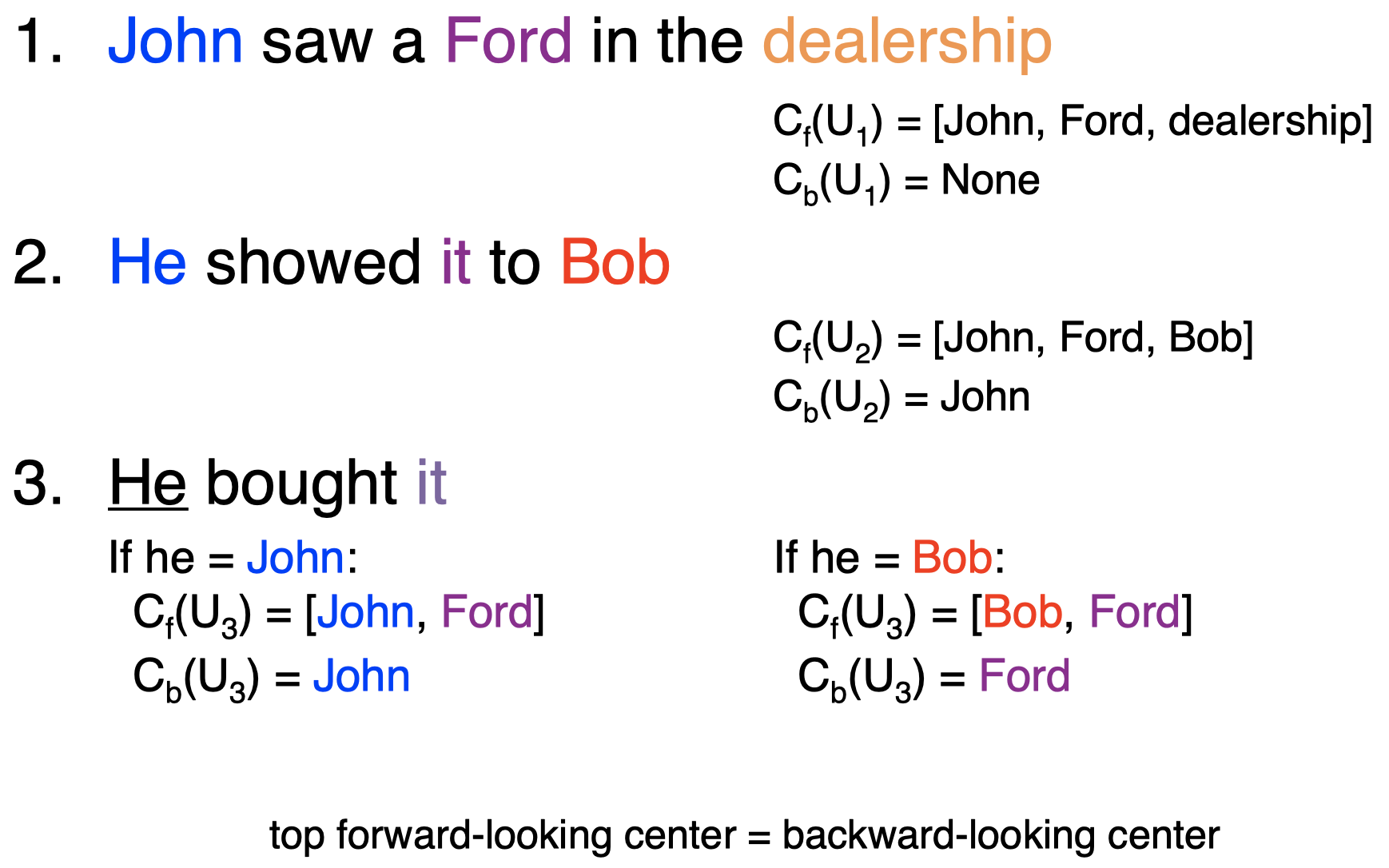• 对于第一个句子，我们计算其前向中心和后向中心：
• $C_f(U_1)=[\textit{John, Ford, dealership}]$
• $C_b(U_1)=\textit{None}$

因为这是第一个句子，前面没有其他句子了。

• 对于第二个句子，我们计算其前向中心和后向中心：
• $C_f(U_2)=[\textit{John, Ford, Bob}]$
• $C_b(U_2)=\textit{John}$

因为前面第一个句子的前向中心中 “$John$” 排名最高，并且该实体在当前句子的前向中心中也出现过了。

• 对于第三个句子，我们计算其前向中心和后向中心： 这里，代词 “$\textit{He}$” 具有歧义性，可能指代前面的 “$John$” 或者 “$Bob$”。
• 如果代词 “$\textit{He}$” 在这里指代前面的 “$John$”，我们计算其前向中心和后向中心：
• $C_f(U_3)=[\textit{John, Ford}]$
• $C_b(U_3)=\textit{John}$
• 如果代词 “$\textit{He}$” 在这里指代前面的 “$Bob$”，我们计算其前向中心和后向中心：
• $C_f(U_3)=[\textit{Bob, Ford}]$
• $C_b(U_3)=\textit{Ford}$

这里，中心算法会选择实体 “$John$” 作为代词 “$\textit{He}$” 的参照。因为在这种情况下，前向中心 $C_f(U_3)$ 中排名最高的实体和后向中心 $C_b(U_3)$ 的结果是相匹配的；而当我们选择 “$Bob$” 作为 “$\textit{He}$” 的参照时，我们会发现前向中心 $C_f(U_3)$ 中排名最高的实体是 “$Bob$”，而计算得到的后向中心 $C_b(U_3)$ 的结果是 “$Ford$”，二者不相匹配。

### 4.8 有监督指代消解

• 为照应语/先行词对构建一个二分类器。
• 将先行词的约束和偏好转换为特征：
• 二进制特征可以用于数量/性别约束
• 先行词在文本中的位置
• 包含有关先行词类别的特征
• 如果我们有充足的数据量，我们可以利用有监督机器学习来近似中心算法。
• 而且还可以很容易囊括一些和趋势（而非规则）相关的特征。
• 例如：重复（repetition），排比（parallelism）等。

### 4.9 指代消解工具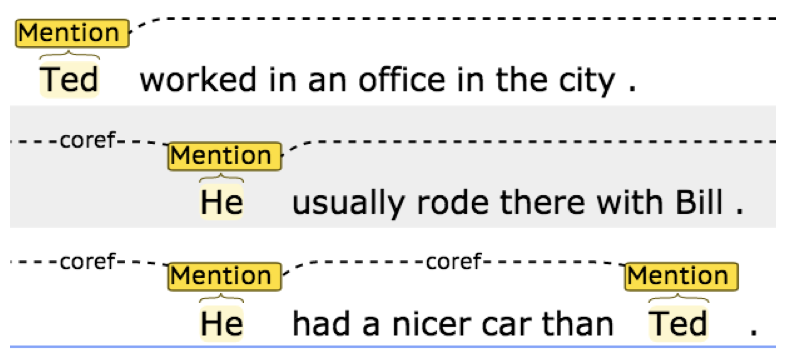• 基于规则的系统效果非常好
• 比学习模型要快得多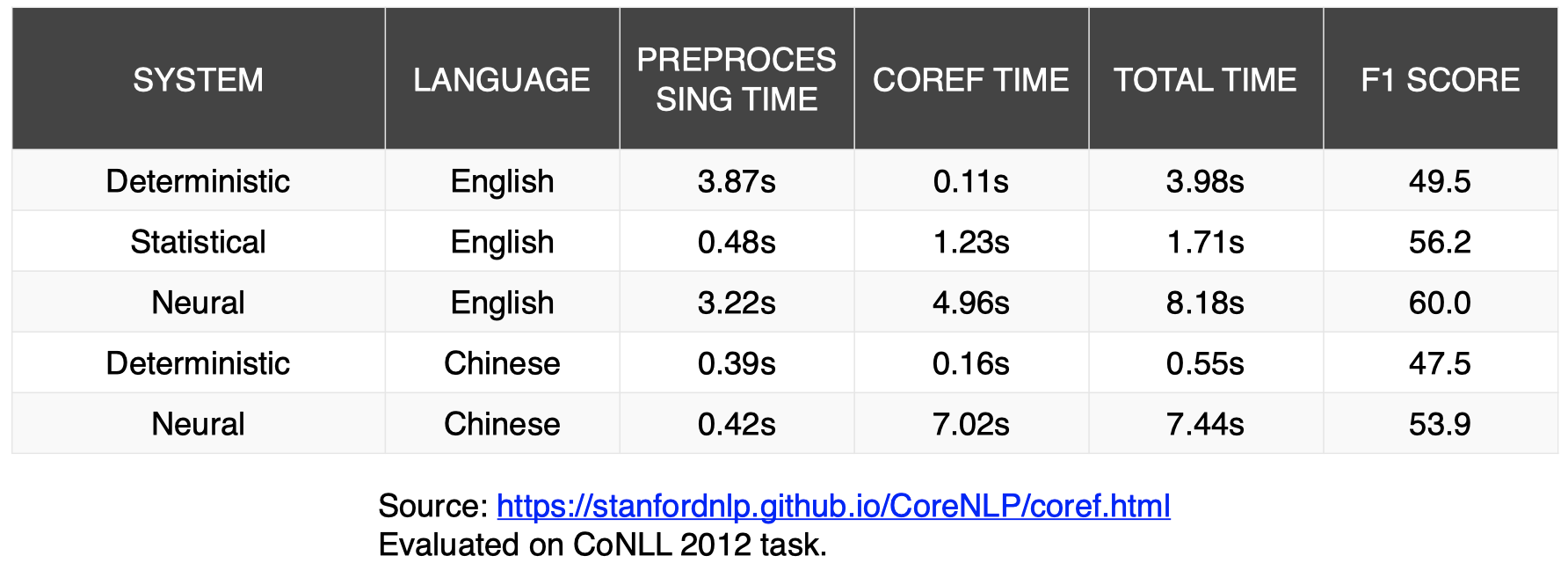### 4.10 指代消解的动机## 5. 总结

• 对于许多任务，考虑上下文要比考虑句子本身更重要。
• 传统上，许多流行的 NLP 应用程序的关注点都在句子层面（例如机器翻译），但是这种情况已经开始改变……

## 6. 扩展阅读

• E18, Ch 16本作品采用知识共享署名-非商业性使用-相同方式共享 4.0 国际许可协议进行许可。 欢迎转载，并请注明来自：YEY 的博客 同时保持文章内容的完整和以上声明信息！